Rhea answered 5/11 in the questions correctly and Precious answered 7/11 of it correctly. If each problem is worth the same amount, who got the higher score?

Result

x = (Correct answer is: Precious)### Step-by-step explanation: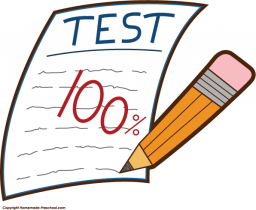Did you find an error or inaccuracy? Feel free to write us. Thank you!Tips to related online calculators
Need help to calculate sum, simplify or multiply fractions? Try our fraction calculator.

## Related math problems and questions:

• QuizTested student answered correctly on a 4/ 8 questions. Wrong answers was 16. How many questions answered?
• Nathan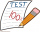Nathan is playing a trivia game. Each correct answer is worth 2 ½ points, and each incorrect answer is worth -¼ points. If he gets 35 questions correct and 32 questions incorrect, how many points does he have? A. 87.5 B. 79.5 C. 75 D. 8
• Roma ateRoma ate 2/5 of a cake while Somya ate 3/7 of the same cake. Who ate more and by how much?
• The math testThe math test contains 20 problems. For each correctly solved problem, the solver gets 3 points, for each incorrectly solved or unsolved problem, 2 points are deducted. Ondrej got 25 points. How many problems did he solve correctly?
• TorqueTorque and Mari each multiplied 1/8 inch times 5/8 inch. Tartaric 5/8 squares point inches. And Marie got 5/64 squared thought inches tall. Which student found a corrupt area?
• Comparing and sortingArrange in descending order this fractions: 2/7, 7/10 & 1/2
• Pie division5/8 of a pie divide 6 pieces. Each friend got 1/6.  What fraction  of the whole pie does each person receive?
• WithdrawalIf I withdrew 2/5 of my total savings and spent 7/10 of that amount. What fraction do I have in left in my savings?
• Math testObelix filled a mathematical test in which he answered 25 questions. For every correct answer, he received 5 points, for each bad answer he had 3 points deducted. Obelix gained 36% of all points in the test. How many questions did he solve correctly?
• TV competitionIn the competition, 10 contestants answer five questions, one question per round. Anyone who answers correctly will receive as many points as the number of competitors answered incorrectly in that round. After the contest, one of the contestants said: We
• Rope cuttingShane has a piece of rope that is 7 4/5 units long. If he cuts it into pieces that are each 3/5 of a unit long, how many pieces does he have?7 is added to the sum of 4/5 and 6/7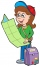Leo hiked 6/7 of a kilometer. Jericho hiked 2/3 kilometer. Who covered a longer distance? How much longer?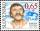The land is worth 33295 CZK. 1st owner has 5/15, 2nd owner has 4/15, 3rd owner has 1/5 4th owner has 1/5. How much CZK each of them will receive?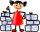At the paper collecting contest gathered Franta 2/9 ton, Karel 1/4 ton, and Patrick 19/36 tons of paper. Who has gathered the most and the least?Sandy, John and Marg baked pies for the Bake Sale. Sandy cut his pies into 6ths, John but his into 8ths, and Marg cut hers into quarters. Sandy sold 11/6, John sold  1 3/8 pies, and Marg sold 9/4 pies. Who sold the most pies? Who sold the fewest?The answer will be given 8 marks, and the wrong answer will be deducted 3 marks. The total number of marks obtained by the school is 36. If Petra answered six questions correctly,   how many questions were answered incorrectly by the student from the scho